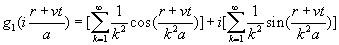Appendix C

Zeta-exponential function

As we know the exponential function is:The Riemann Zeta function is: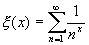Combine the exponential function and zeta function, and then we can have a new function, which we temporally called the zeta-exponential function as follows: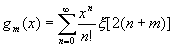Then we will get: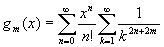Thus: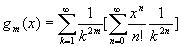Thus: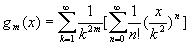Thus: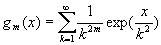Thus, we have: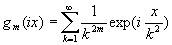The above equation is the
Fourier serial of the complex zeta-exponential function.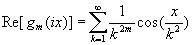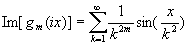As we know: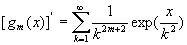Thus:As we know for the exponential function, we have: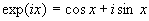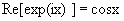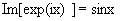Similar to the exponential function, the complex zeta-exponential function has the following relationship: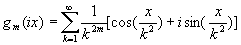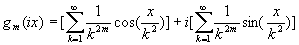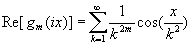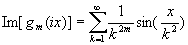for m =1 and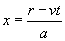, thus we have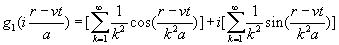for m =1 and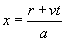, thus we have